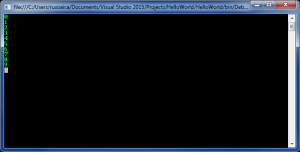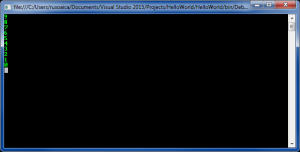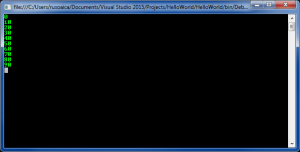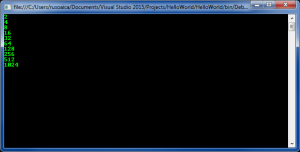Saturday, September 23, 2023 21:54

## For loop

For loop is a kind of repeating code construct which is a bit more complex than the previous types of loops we learned so far. On the other hand, they can solve more complicated tasks, with less code involved.

The syntax of the For instruction is the following:

When the program repeats (cycles) the instructions for a certain number of times, you will usually use a variable called control variable, which indicates how many times you executed the instructions. So, the For loop contains four sections. start_value section initializes our control variable with a default value, which most of the times is 0. The next section, end_condition usually tests the control variable value to see if the instructions were ran the desired number of times. increment_value section usually increments or decrements our control variable by 1 (depending on the behavior we are expecting) or any other value we want, each time the loop runs the instructions. Finally, the fourth section of the For loop are the loop block, in which we place the instruction or the instructions we want to be executed whenever the loop cycles.

Lets take the simplest example for demonstrating the usage of the For loop:

The output on the console will be:In our example, we declared an integer variable (our control variable) called counter and initialized it to the default value of 0. Next, we specified that we want the loop to be executed only if our counter variable’s value is less than 10. Finally, we instructed it to increment our counter variable by 1 on each cycle of the loop.

So, we initialized a variable called counter to the value 0. The For loop executed the instructions inside the loop block and increments the value of counter by 1. After that, it checks the condition: is counter less than 10? If yes, run the instructions again, increment the variable again, perform the check of the value again, etc, until the check of our counter variable’s value determines that it’s value was incremented to 10, which will return False when we check if our variable is less than 10.

As i said before, it is not imperative that we increment our variable. we can also decrement it:

Which will display:In this case, the loop will perform the same way as in our previous case, with three differences: first, we initialized our variable as 9, not 0; second, we specified the ending condition of our loop to be when our counter variable is greater than or equal to 0; third, we instructed our variable to decrement by 1 each loop. Basically, the program will now count down from 9 to 0.

We could also specify a different increment factor:

You can already figure it out. We told our program to increment our control variable by 10 on each cycle, until the value gets equal or greater than 100:We can also use any other mathematical operation, for instance multiplication:

which will make us count in multiplication steps by 2:You should also understand the scope  of our control variable (which sections of the code is it visible and accessible). Following example will demonstrate this aspect:

So, when we declare our control variable in our For loop, it is only visible inside that loop. Happily, we can use an external variable, and simply omit to declare one in our loop:

From the above code, you can see that since we declared our num variable outside the for loop, it is visible and accessible from inside and outside our loop. Also you can notice that since we declared it already outside the for loop, we can omit its declaration inside our loop; however, we still need to place the ; character.

The declaration part is not the only thing we can omit in a for loop. For instance, in the following example, we are declaring our control variable outside the loop, and increment it inside the For loop body:

Hack, we can even declare a For loop without any parameters, like so:

In this case, we will get an infinite loop, since there is no condition to end it, and the program will continue to print “Iterated!” until the end of times (or until you close it 😉 )

Finally, you can specify multiple variable controls and conditions for a single For loop. For instance:

The concepts explained in this lesson are also shown visually as part of the following video:

EXERCISES
1. Write a program that prints on the console the numbers from 1 to N. The number N should be read from the standard input.

Solution

Guidelines: Use a `for`-loop.

2. Write a program that prints on the console the numbers from 1 to N, which are not divisible by 3 and 7 simultaneously. The number N should be read from the standard input.

Solution

Guidelines: Use a `for`-loop and the operator % for finding the remainder in integer division. A number is divisible by 3 and 7 simultaneously exactly when `(num % (3*7) == 0)`.

3. Write a program that reads from the console a series of integers and prints the smallest and largest of them.

Solution

Guidelines: First read the count of numbers, for example in a variable `n`. Then consequently enter n numbers with one `for` loop. While entering each new number, save in two variables the smallest and the largest number until this moment. At the start initialize the smallest and the largest number with `Int32.MaxValue` and `Int32.MinValue`respectively.

4. Write a program that reads from the console number N and print the sum of the first N members of the Fibonacci sequence: 0, 1, 1, 2, 3, 5, 8, 13, 21, 34, 55, 89, 144, 233, 377, etc.

Solution

Guidelines: Fibonacci numbers start from 0 and 1, each additional is obtained as the sum of the previous two. You can find the first `n` Fibonacci numbers with a `for`-loop from 1 to `n`, and at each iteration calculate the next number by using the previous two (which you will keep in two additional variables).

Tags: ,